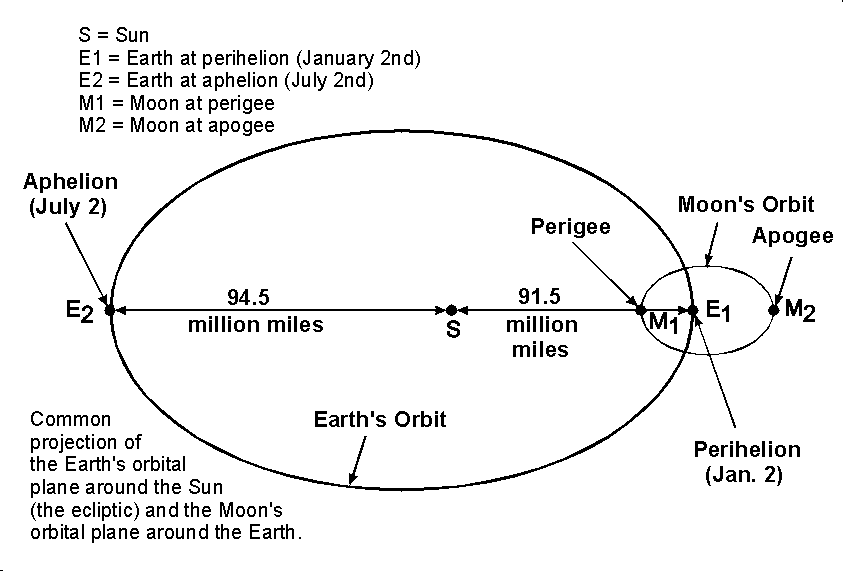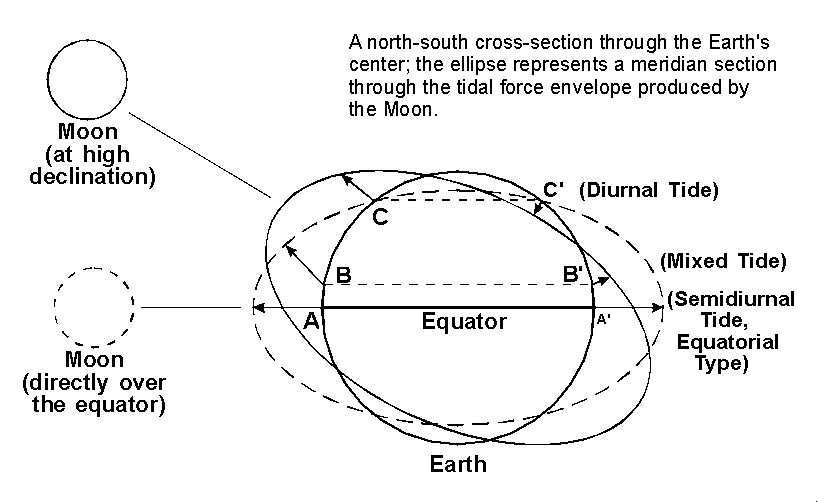### Chapter 4 - Variations in the Range of the Tides: Tidal Inequalities

As will be shown in Fig. 6, the difference in the height, in feet, between consecutive high and low tides occurring at a given place is known as the range. The range of the tides at any location is subject to many variable factors. Those influences of astronomical origin will first be described.

1. Lunar Phase Effect: Spring and Neap Tides. It has been noted above that the gravitational forces of both the moon and sun act upon the waters of the earth. It is obvious that, because of the moon's changing position with respect to the earth and sun (Fig. 3) during the monthly cycle of phases (29.53 days) the gravitational attraction of moon and sun may variously act along a common line or at changing angles relative to each other.

When the moon is at new phase and full phase (both positions being called syzygy) the gravitational attractions of the moon and sun act to reinforce each other. Since the resultant or combined tidal force is also increased, the observed high tides are higher and low tides are lower than average. This means that the tidal range is greater at all locations which display a consecutive high and low water. Such greater-than-average tides resulting at the syzygy positions of the moon are know as spring tides - a term which merely implies a "welling up" of the water and bears no relationship to the season of the year.

At first- and third-quarter phases (quadrature) of the moon, the gravitational attractions of the moon and sun upon the waters of the earth are exerted at right angles to each other. Each force tends in part to counteract the other. In the tidal force envelope representing these combined forces, both maximum and minimum forces are reduced. High tides are lower and low tides are higher than average. Such tides of diminished range are called neap tides, from a Greek word meaning "scanty".

2. Parallax Effects (Moon and Sun). Since the moon follows an elliptical path (Fig. 4), the distance between the earth and moon will vary throughout the month by about 31,000 miles. The moon's tide-producing force acting on the earth's waters will change in inverse proportion to the third power of the distance between the earth and moon, in accordance with the previously mentioned variation of Newton's Law of Gravitation. Once each month, when the moon is closest to the earth (perigee), the tide-generating forces will be higher than usual, thus producing above-average ranges in the tides. Approximately two weeks later, when the moon (at apogee) is farthest from the earth, the lunar tide-raising force will be smaller, and the tidal ranges will be less than average. Similarly, in the earth-sun system, when the earth is closest to the sun (perihelion), about January 2 of each year, the tidal ranges will be enhanced, and when the earth is farthest from the sun (aphelion), around July 2, the tidal ranges will be reduced.##### The Lunar Parallax and Solar Parallax Inequalities

FIGURE 4

Both the Moon and the Earth revolve in elliptical orbits and the distances from their centers of attraction vary. Increased gravitational influences and tide-raising forces are produced when the Moon is at position of perigee, its closest approach to the Earth (once each month) or the Earth is at perihelion, its closest approach to the Sun (once each year). This diagram also shows the possible coincidence of perigee with perihelion to produce tides of augmented range.

When perigee, perihelion, and either the new or full moon occur at approximately the same time, considerably increased tidal ranges result. When apogee, aphelion, and the first- or third-quarter moon coincide at approximately the same time, considerably reduced tidal ranges will normally occur.

3. Lunar Declination Effects: The Diurnal Inequality. The plane of the moon's orbit is inclined only about 5o to the plane of the earth's orbit (the ecliptic) and thus the moon monthly revolution around the earth remains very close to the ecliptic. The ecliptic is inclined 23.5o to the earth's equator, north and south of which the sun moves once each half year to produce the seasons. In similar fashion, the moon, in making a revolution around the earth once each month, passes from a position of maximum angular distance north of the equator to a position of maximum angular distance south of the equator during each half month. (Angular distance perpendicularly north and south of the celestial equator is termed declination.) twice each month, the moon crosses the equator. In Fig. 5, this condition is shown by the dashed position of the moon. The corresponding tidal force envelope due to the moon is depicted, in profile, by the dashed ellipse.##### The Moon's Declination Effect (Change in Angle With Respect to the Equator) and the Diurnal Inequality; Semidiurnal, Mixed, and Diurnal Tides

FIGURE 5

A north-south cross-section through the Earth's center; the ellipse represents a meridian section through the tidal force envelope produced by the Moon.

Since the points A and A' lie along the major axis of this ellipse, the height of the high tide represented at A is the same as that which occurs as this point rotates to position A' some 12 hours later. When the moon is over the equator - or at certain other force-equalizing declinations - the two high tides and two low tides on a give day are at similar height at any location. Successive high and low tides are then also nearly equally spaced in time, and occur twice daily. (See top diagram in Fig. 6.) This is known as semidiurnal type of tides.

However, with he changing angular distance of the moon above or below the equator (represented by the position of the small solid circle in Fig. 5) the tidal force envelope produced by the moon is canted, and difference between the heights of two daily tides of the same phase begin to occur. variations in the heights of the tides resulting from the changes in the declination angle of the moon an in the corresponding lines of gravitational force action give rise to a phenomenon known as the diurnal inequality.

In Fig. 5, point B is beneath a bulge in the tidal envelope. One-half day later, at point B' it is again beneath the bulge, but the height of the tide is obviously not as great as at B. This situation gives rise to a twice-daily tide displaying unequal heights in successive high or low waters, or in both pairs of tides. This type of tide, exhibiting a strong diurnal inequality, is known as a mixed tide. (See the middle diagram in Fig. 6.)

Finally, as depicted in Fig. 5, the point C is seen to lie beneath a portion of the tidal force envelope. One-half day later, however, as this point rotates to position C', it is seen to lie above the force envelope. At this location, therefore, the tidal forces present produce only one high water and one low water each day. The resultant diurnal type of tide is shown in the bottom diagram of Fig. 6.##### Principal Types of Tides

FIGURE 6

Showing the Moon's declinational effect in production of semidiurnal, mixed, and diurnal tides.

Chapter 5 - Factors Influencing the Local Heights and Times of Arrival of the Tides